# EdPlace's GCSE Home Learning Maths Lesson: Simplifying Algebraic Fractions

Looking for short lessons to keep your child engaged and learning? Our experienced team of teachers have created English, maths and science lessons for the home, so your child can learn no matter where they are.  And, as all activities are self-marked, you really can encourage your child to be an independent learner.

Get them started on the lesson below and then jump into our teacher-created activities to practice what they've learnt. We've recommended five to ensure they feel secure in their knowledge - 5-a-day helps keeps the learning loss at bay (or so we think!). Are they keen to start practising straight away? Head to the bottom of the page to find the activities.

Now...onto the lesson!

Key Stage 4 Statutory Requirements for Maths
GCSE students must be able to simplify and manipulate algebraic fractions by collecting like terms, taking out common factors and factorising expressions

# Why Learn About Algebraic Fractions?

Ever sat down to dinner and found your family discussing maths homework?  Not a problem... as long as you understand the words your child is using, but how do you feel about the two words... ‘algebraic fractions’? For most of us, the last time we heard this was a fair way back, which makes answering your child's question...‘why do we have to learn this anyway?’ all the more challenging to answer. Well, if your child has ever wanted a career in Aerospace, as an engineer, or designer you can tell them that they need to be able to use and simplify algebraic fractions. Bridge designers use algebraic fractions to ensure their designs are structurally safe and opticians also use algebraic fractions when working out lens prescriptions... interesting huh!?

So, take a deep breath as we look at how to put this concept into practice. As long as your child can multiply, divide and simplify then they already have the necessary skills! Algebraic fractions are an extension of these basic skills and a great excuse to play around with that dreaded algebraic x!
We're confident that by by following this step-by-step approach your child will be able to:

1) Understand the different skills required to be able to manipulate algebraic fractions

2) Describe how to simplify an algebraic fraction

3) Apply this knowledge independently

## Step 1 - Back to Basics

Before we get started, we need to make sure your child understands the following key concepts.

Simplify – this means to make things as easy as you can at the beginning of your working out

Common factors – to look for values which can be connected with one another

Factors – numbers which divide into another number equally e.g. the factors of 24 are 1, 2, 3, 4, 6, 12 and 24

Factorise – to put values into brackets

We will work through these key terms one at a time in the following steps.

## Step 2 - Simplify an Expression

Simplify 7/28

This just means to look out for common factors. Is there a number that can divide into both 7 and 28?  Each time we must look for the largest number. 7 can be divided by itself once, and it goes into 28, four times. Therefore 7/28 simplifies to 1/4.

Simplify 4x/2

This is no different! We can see that 2 will divide into itself once and into 4 twice. There's nothing in common with x, so we leave it alone. So this fraction would simplify to 2x/1. However, because the fraction is over 1 we tend to ignore this and write it as just 2x.

It's worth noting that 5x² simplifies to 1x² in algebra because the number in front of the letters is ignored. Therefore, 35x²/7 is written as x²/7

Top Tip: Don’t let your child worry that there are no numbers in a fraction as this doesn’t change the method. If there are two x’s on the top of the fraction and one on the bottom, the x is the common factor. If you take one x off the bottom and one off the top you are just left with x.

## Step 3 - Factorise Before Simplifying

Factorising helps to find things in common which are not obvious straight away. It means putting brackets into an expression, which allows your child to see where the simplification is. For example, look for something that is common to place outside of the bracket in the fraction below: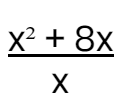As there is only one thing in the bottom half of the fraction it can be ignored for now. You have two x’s on one side of the plus sign and one on the other. So, x is common and is placed outside of the bracket. You write x (x + 8) and are left with x + 8 to go inside the bracket.

For clarity, look at it this way: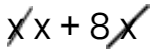Now we have to look at the bottom row and simplify as in the example above.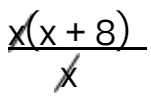The answer is x + 8.

## Step 4 - Factorising the Top and Bottom

Simplify: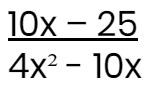Make sure your child deals with one line at a time. Quite often students look at the whole thing and freak out, which might make you do the same!

1) In the top line, 10x – 25, The common number here is 5, which we place outside of the bracket.

2) 5 divides into 10 twice and 25 five times giving 5(2x – 5)

3) On the bottom line, 4x² - 10x, the common factors here are x and 2 which is placed outside of the bracket

4) 2 divides into 4 twice and 10 five times giving 2x(2x – 5)

5) Now put the two together and simplify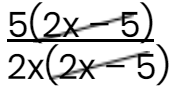which leaves us with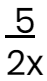Let's try some more challenging factorisation. Hold tight... we're going in for the big one!

Simplify: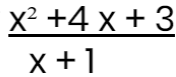Luckily, the bottom has already been factorised. However, the top looks a bit different from what your child has already mastered.

1) Because there is an x² on the top line, you are going to need two brackets and to put one x in each (x)(x).

This next part can become a little tricky, but it's only an extension of basic addition and multiplying.

2) Encourage your child to look for two numbers that add up to get the middle number (4) but also multiply to get the last number (3). So, 2 + 2 doesn’t work because although they add up to 4, they don’t multiply to 3. Looking again we can see that 3 + 1 = 4 and 3 x 1 = 3. Bingo!

3) We now put these numbers into the brackets (x + 1)(x + 3). As there are only addition signs in the expression it doesn’t matter which number goes into which bracket (when there are minus signs involved it will matter).

Rewrite the expression as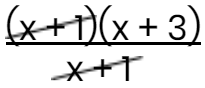and simplify.

Why not try tackling some more practice questions together?

Simplify the following fractions:

1)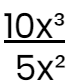2)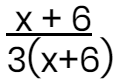3)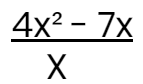4)5)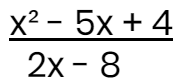## Step 5 - Give it a go...

Now that you’ve learnt to simplify algebraic fractions, see if your child can apply their knowledge to the following 5 activities. Have them complete them in the order listed below. All activities are created by teachers and automatically marked.

Plus, with an EdPlace subscription, we can automatically progress your child at a level that's right for them. Sending you progress reports along the way so you can track and measure progress, together - brilliant!

1) 2x

2) 3

3) Factorising the top gives x(4x - 7) which in turn simplifies to 4x - 7

4) Factorising gives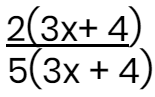which simplifies to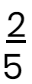5) Factorising top and bottom gives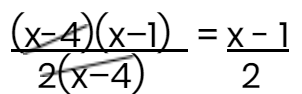### Keep going! Looking for more activities, different subjects or year groups?

Click the button below to view the EdPlace English, maths, science and 11+ activity library